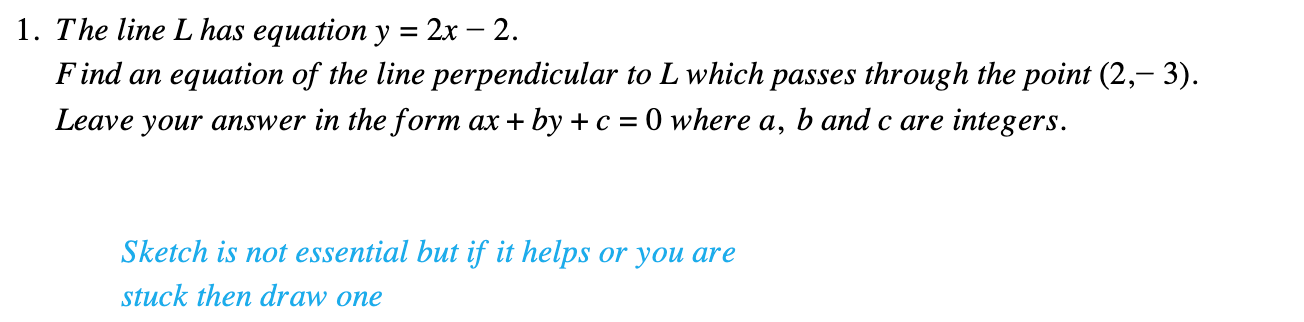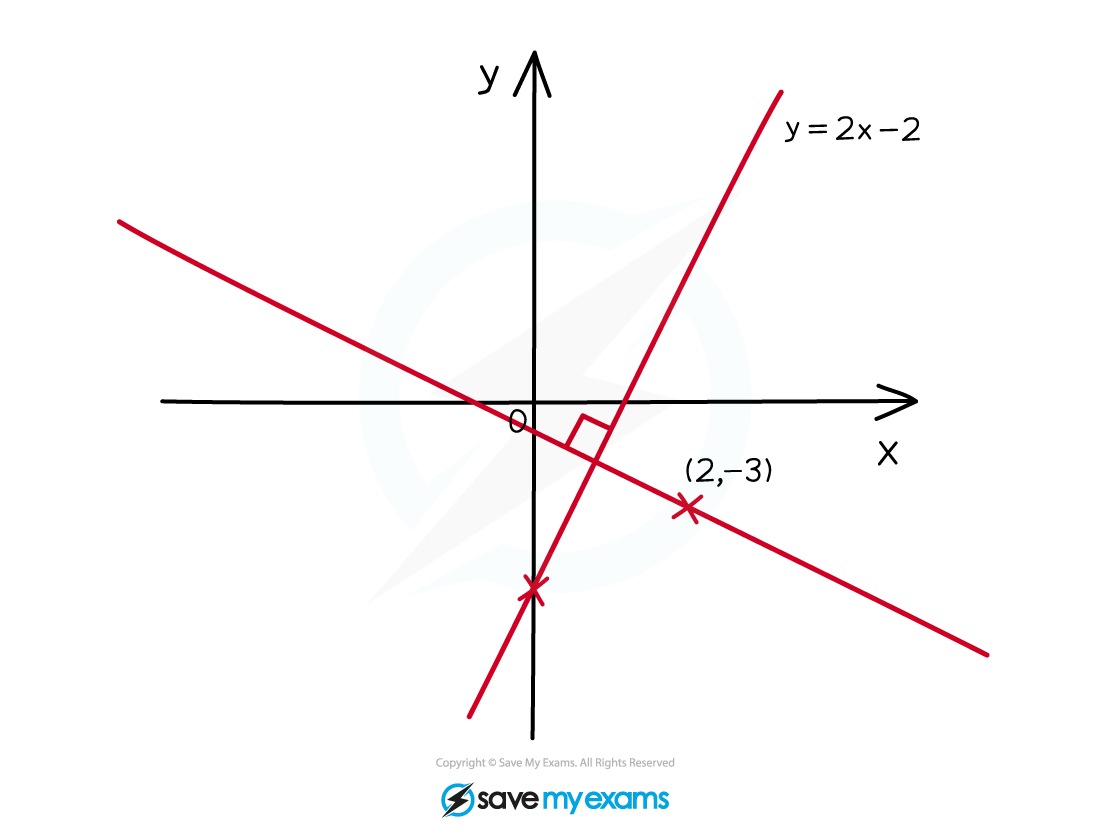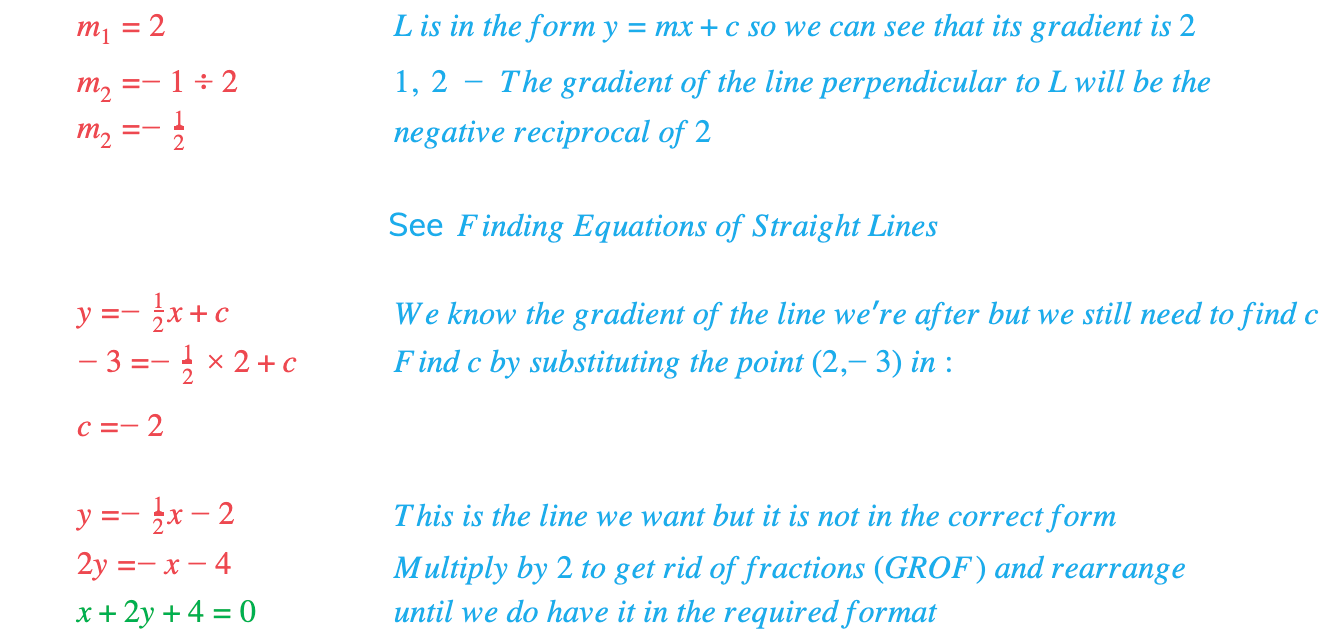# Edexcel IGCSE Maths 复习笔记 3.7.1 Perpendicular Lines

Edexcel IGCSE Maths 复习笔记 3.7.1 Perpendicular Lines

#### What are perpendicular lines?

• PERPENDICULAR LINES do meet each other and where they do the two lines form a right angle – ie they meet at 90°

#### What’s the deal with perpendicular gradients (and lines)?

• Before you start trying to work with perpendicular gradients and lines, make sure you understand how to find the equation of a straight line – that will help you do the sorts of questions you will meet
• Gradients m1 and m2 are PERPENDICULAR if m1 × m2 = -1
• We can use m2 = -1 ÷ m1 to find a perpendicular gradient

(This is called the NEGATIVE RECIPROCAL)If in doubt, SKETCH IT!

#### Worked Example